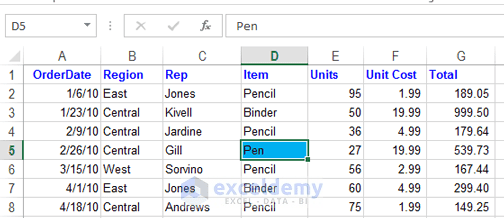# What is spreadsheet and how it works

A spreadsheet is basically a worksheet which is divided into rows and columns to store data; data from business inventories, income-expense report, and debit-credit calculations. Today’s business world uses electronic spreadsheets in the place of antiquated paper-based worksheets.

You can change the value stored in a spreadsheet cell. Changing the value in an old-fashioned paper-based worksheet was toilsome as it created tedious manual recalculation.

1. Rows – Rows are typically labeled using numbers (1, 2, 3, 4, …).
2. Columns – Columns are typically labeled using letters (A, B, C, D, …)
3. And Cells – The intersection of a column and a row makes a cell in a spreadsheet.

In a spreadsheet, the column is the vertical space that is going up and down the spreadsheet. The highlighted part of the following spreadsheet is a column and it is labeled B.

In a spreadsheet, the row is the horizontal space that is going across the spreadsheet. The highlighted part of the following spreadsheet is a row and it is labeled number 5.

In a spreadsheet, a cell is a place where a column and a row intersects. Every cell is assigned an address with its column letter and row number. Remember column letter will come first and row number will be placed second in a cell’s address. An Excel 2013 spreadsheet is made up of more than 17 billion cells.Spreadsheet Cell. Highlighted space is a cell created by the cross-section of column D and row 5, cell address D5.

### Last cell in a spreadsheet

In an Excel 2013 spreadsheet, column letters range from A to XFD, total 16,384 columns and row numbers range from 1 to 10, 48,576. The last cell address is then XFD1048576 in an Excel spreadsheet.

### The data types you can store in the cells

A modern spreadsheet is made up of a huge number of cells. A Microsoft’s Excel 2013 spreadsheet holds more than 17 billion cells. A cell holds three basic types of data:

Read More: what is cell in excel definition

• Numeric value
A numeric value can be a general number, a scientific number, date, time, or a formatted number. Dates (such as Feb-26-2013) or times (such as 3:24 a.m.) are considered as numeric values.
• Text
Texts can be labels for values, headings for columns, or instructions about a spreadsheet. Text if begins with numbers or text with numbers is still considered the text. For example ‘6/1 Block C’ is text ‘as well as Block C, 6/1’.
• Formula
Formulas are what make a spreadsheet a spreadsheet. When you enter a formula into a cell, the formula’s result appears in the cell. Formulas can be simple mathematical expressions, or they can use some of the powerful functions that are built into Excel.

### What is spreadsheet’s invisible drawing layer

A spreadsheet can also hold charts, diagrams, pictures, buttons, and other objects. But these objects aren’t contained in cells. Instead, they reside on the worksheet’s drawing layer, which is an invisible layer on top of each worksheet.

Excel is the most used spreadsheet program in the world and industry standard. There are other Excel programs but Excel is the most popular.

#### Kawser

Hello! Welcome to my Excel blog! It took me some time to be a fan of Excel. But now I am a die-hard fan of MS Excel. I learn new ways of doing things with Excel and share here. Not only how to guide on Excel, but you will get also topics on Finance, Statistics, Data Analysis, and BI. Stay tuned!